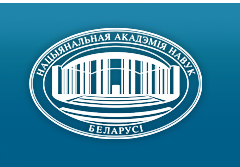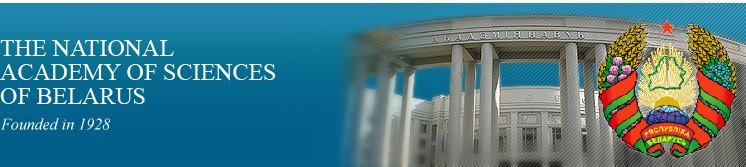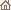Home News About Academy Academy Activities Academy Membership Organizations Publications Conferences Contacts Site Map

# Proc. of the Natl. Academy of Sciences of Belarus, Ser. Phys.-Math. Sci., 2011, No.4/ Publications / Scientific Journals

### Number 4, 2011## CONTENTS

### MATHEMATICS

Zhuk E. E.
Maximum likelihood decision rule stability under violation of the assumption of class equiprobability. pp. 5-10.

Summary: The maximum likelihood decision rule stability is investigated under violation of the assumption of class equiprobability. The risk bias is analytically evaluated by the risk asymptotic expansion method. The obtained results are illustrated for the well-known Fisher model.

Demenchuk A. K.
Control of a spectrum of irregular periodic oscillations of linear systems with a block triangular represetation. pp. 11-16.

Summary: We consider the linear control periodic system with reverse communication. It is supposed that the average matrix coefficient is a block triangular representation in the noncritical case. The control problem of a spectrum of irregular oscillations is solved.

Malyutin V. B.
Method for approximate evaluation of functions of matrix variables. pp. 17-23.

Summary: The method for approximate evaluation of the functions of matrix variables is proposed. Approximation of the function by an algebraic-type interpolation matrix multinomial with two matrix variables is used in this method. The application of the proposed method for approximate evaluation of some chronological ordered exponents and matrix-valued integrals of cylinder functions is considered.

Uaziz A. H., Yablonski A. L.
Limit behavior of the solutions to the finite-difference equations with the polynomial approximation. pp. 24-32.

Summary: The finite-difference equation, which associates the differential equation with generalized coefficients, is studied. The limit behavior of the solution to this equation in the case of polynomial approximation is investigated.

Grytsuk E. V.
Local properties of the solutions of higher analogs of the first Painleve equation. pp. 33-41.

Summary: The local properties of solutions of higher analogs of the first Painleve equation in the neighborhood of a movable pole are investigated.

Andreeva D. P., Skiba A. N.
S-quasinormal subgroups in maximal short chains of finite groups. pp. 42-47.

Summary: The article is devoted to a description of properties of finite groups, in which every maximal chain of length 3 contains a proper S-quasinormal subgroup. In particular, the article contains the theorem, proving that the group G is a widening of a nilpotent group with the help of the Abelian group, the exponents of which are cube-free.

Korzyuk V. I., Kozlovskaya I. S., Sheika J. V.
Solution of the Cauchy problem for the telegraph equation by the characteristic method. pp. 48-54.

Summary: A general solution of the telegraph equation and a classical solution of the Cauchy problem for homogeneous and inhomogeneous telegraph equations were obtained. The convergence method was used to obtain a general solution of the telegraph equation. The solution of the inhomogeneous telegraph equation was derived by the Duhamel method. The offered approach to the solution of the Cauchy problem for the telegraph equation allows one to solve mixed problems for the telegraph equation both in cylindrical and non-cylindrical domains.

Budzko D. A.
Stability analysis of the equilibrium solutions of the restricted four-body problem. pp. 55-59.

Summary: In the present article we have investigated the stability in Liapunov's sense of equilibrium solutions in the circular planar restricted four-body problem formulated on the basis of Lagrange's triangular solutions.

Nifagin V. A., Bubich M. A.
Asymptotic expansion method in the theory of elasto-plastic cracks. pp. 60-66.

Summary: The expansion method in the parameter loading is applied to solve the problem of a crack in the common form of the elastic-plastic plane with power-hardening. The system of resolving equations in increments is reduced to a recursive sequence of boundary eigen value problems for nonlinear differential operators. A variant of the numerical-analytical shooting method is used for estimation of the stress-strain state in the neighborhood of the crack tip. To investigate the influence of plastic properties of the material to the fracture to be produced, the numerical analysis of stress fields near the singular point is made.

### PHYSICS

Kudryashov V. V., Baran A. V.
Rashba and Dresselhaus spin-orbit interactions of equal strength in the circular quantum dot. pp. 67-70.

Summary: The exact solutions of the Schrodinger equation are obtained for an electron in two-dimensional circular semiconductor quantum dot in the presence of the Rashba and Dresselhaus spin-orbit interactions of equal strength. Confinement is simulated by a realistic potential well of finite depth. The dependence of energy levels on a strength of spin-orbit interaction and a depth of a potential well is presented.

Baryshevsky V. G., Gurinovich А. А.
Radiative instability of a relativistic electron beam moving in a photonic crystal. pp. 71-78.

Summary: The radiative instability of an electron beam moving in a finite photonic crystal is analyzed and the dispersion equation for this case is obtained. General rules for deriving the dispersion equation in a finite photonic crystal are stated. They are also valid for description of the electromagnetic wave instability in a nonlinear finite photonic crystal.

Tsyanenka D. A.
Tensor field equations of first-order in the non-Euclidean space-time. pp. 79-84.

Summary: A new method to the description of the first-order wave equations in the non-Euclidean space-time is presented. This approach is based on the technique of generalized Kronecker symbols. The extension of the Dirac-Kahler equation to the case of the curved space-time is given. Possible modifications of equations by the introduction of symmetrical additions to the an-tisymmetrical tensors with the use of a metric tensor are investigated.

Ovsiyuk E. M.
Solutions of the Dirac equation in analogs of uniform magnetic and electric fields in the spherical Riemann space. pp. 85-92.

Summary: The concept of a uniform electric field is determined in a 3-dimensional sphere as a solution of covariant Maxwell equations. This electric field is invariant under transverse shifts in the spherical space and not invariant under longitudinal shifts. Exact solutions of the Dirac equation in the presence of the magnetic and electric fields oriented along one the same direction, are constructed. The topology of the curved space model and the presence of the external electric field lead to quantization of the motion of the Dirac particle along the z axis, additional to quantization of the transverse motion of the particle due to the uniform magnetic field.

Ryabushko A. P., Zhur T. A., Boyarina I. P.
Motion of a body in the gravitational field with light pressure in the post-Newtonian approximation of the general relativity. pp. 93-97.

Summary: In the post-Newtonian approximation of the general relativity the energy integral, the orbital angular momentum integral, and the equation of the orbit of a test body with light pressure have been derived and discussed. Several relativistic effects are predicted. Some numerical values of these effects are given.

Korshunov F. P., Marchenko I. G., Zhdanovich N. E.
Electrical characteristics of Si p-n-structures locally irradiated with fast electrons. pp. 98-103.

Summary: The investigation of the influence of local irradiation with fast electrons (4 MeV) forming zones of enhanced recombination (ZER) in the base region of p+-n-n+-structures on the basic electro-physical parameters of silicon diodes (with forward currents up to 600 A) has been fulfilled in this work. It is shown that irradiation of separate parts of the large area diode structure in comparison with complete irradiation of the whole structure improves the trade-off of reverse recovery time (trr) and energy losses in the conducting state (UF) at simultaneous decreasing of diode reverse current (IR) temperature sensitivity. The dependence of trr, UF and IR trade-off in locally irradiated structures on experiment conditions (ZER size) has been established.

Chobot A. N., Teresko N. V., Mantytskaya O. S., Bespalaya N. Yu.
Magnetic and magnetotransport properties of the Pr0,5Sr0,5Mn1-xCoxO3 (x £ 0,15) system. pp. 104-107.

Summary: The magnetic and magnetotransport properties of Pr0,5Sr0,5Mn1-xCoxO3 are investigated. It is shown that at (x < 0,1) the solid solutions exhibit antiferromagnet-ferromagnet transition while at (x > 0,1) the insulating spin glass state is stabilized. All compositions show a pronounced magnetoresistance effect.

Savchuk G. K., Letko A. K., Klimza A. A.
Dielectric properties of ceramic materials based on zinc titanates. pp. 108-111.

Summary: As a result of investigation, the synthesis conditions of the microvawe ceramic for compact antennas on the basis of the (Zn, Mg)-Ti-O system are studied. The physical and dielectric properties of ceramics are investigated.

Tochitskii T. A., Dmitrieva A. E.
Investigation of the initial stage of forming the structure of Ni/Cu multilayers on cuprum substrates. pp. 112-115.

Summary: The electron-microscopic investigation has shown that the thickness of transition layers, in which the rebuilding texture and the structure of the Ni/Cu multilayers on cuprum substrates occur due to twinning, depends on the cuprum pulse time and is determined by the thickness (40-70 nm) of three nickel bilayers.

### INFORMATICS

Demidenko V. M.
Extension of Monge's conditions to a class of functionals defined on symmetric group. Part I. pp. 116-122.

Summary: Two kinds of generalizations of the classical Monge's conditions for permutational functionals of general form are proposed. These conditions guarantee the constriction of search area of an optimum of a well-known traveling salesman problem up to a set of pyramidal cycles and achievement of a global optimum of a linear assignment problem on identical permutation.

### SCIENTISTS OF BELARUS

Boris Vasilievich Bokut (To the 85th Anniversary of Birthday). pp. 122-124.
Click
here to read this article in Russian (Win 1251)/ Publications / Scientific Journals / Back to Top of Page

Designed and maintained by Dr. Nikolai N. Kostyukovich. Last updated: March 6, 2013
Created with assistance of Mrs. Lyudmila V. Smolentseva
Copyright © 2011-2013 The National Academy of Sciences of Belarus
Copyright © 2011 The Belaruskaya Navuka Publishing House